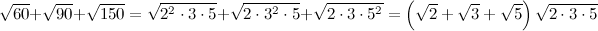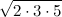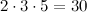## Irrationality

I just started college classes this Thursday. Since I had already taken Calculus III in high school, I was allowed to skip ahead to real analysis. The first day’s assignment had some pretty interesting problems, so I thought I’d share them here.

Preliminaries

Rational numbers are numbers that can be represented as ratio of two integers. Numbers like 1/2, 3/4, 87, and -43/6 and so on. Irrational numbers, then, are numbers which cannot be represented as the ratio between two integers. For a long time it was thought that all things could be measured through rational numbers, and there was no such thing as irrational numbers. To the Pythagoreans, a cult led by the famous mathematician Pythagoras, this belief was sacred. It was so sacred, in fact, that it is rumored that when one of the Pythagoreans, Hippasus, proved that the length of the diagonal of unit square (or the$\sqrt{2}$) was irrational, they bound him up and threw him into the sea. I do not know what methord Hipassus used to prove this, or even whether or not this story is true, but I thought I’d start off by sharing one of my favorite ways I’ve seen this theorem proved.

Proof 1: The Square Root of 2 is irrational

First, assume that the square root of 2 really is rational. That is, it can be written as the ratio of two integers. Thus, we can write

Now comes the interesting part. Remember the fact that all numbers can be represented with a unique prime factorization. So let’s say you know the prime factorization of a, to figure out the prime factorization of$a^2$ you merely multiply all the exponents in a’s prime factorization by two. For example if$a = 2^3*5^2*7$ then$a^2 = 2^6*5^4*7^2$. But since you are multiplying all the exponents by two, they must all end up being even. The same thing goes for the factorization of$b^2$. That means the exponent of “2” in the prime factorization of$b^2$ must be even, but that means that the exponent of 2 in$2b^2$ must be odd. But that means that$2b^2$ can’t possibly be equal to $a^2$ since $a^2$’s factorization has only even exponents. This is a contradiction, and thus we can conclude that$\sqrt{2}$ is indeed irrational.

The great thing about this proof is that it easily extends to any value$\sqrt{n}$ where n  is not a perfect square. Because if n is not a perfect square, it must have an odd exponent in it’s prime factorization, which would mean that$nb^2$ must also have an odd exponent somewhere, which would mean it can’t possibly be equal to$a^2$ as it only has even exponents. Thus, this proof shows that any square root of a number which isn’t a perfect square will result in an in irrational number!

Problems

Now for the problems on my homework assignment. The two problems I want to address are

Prove that$\sqrt{2} + \sqrt{3}$ is irrational
Prove that$\sqrt{2} + \sqrt{3} + \sqrt{5}$ is irrational

If you’d like to, try to prove these on your own before moving on.

The first one is pretty easy if you consider one some pretty obvious facts. If the numbers x and y are rational, then

1.$x+y$ is rational
2.$x-y$ is rational
3.$xy$ is rational

Now, look back at statement 3 . If we let$y = x$ this statement says that if x is rational, then$x^2$ is rational. Which means that if$\sqrt{2} + \sqrt{3}$ is rational, then so is$\left(\sqrt{2} + \sqrt{3}\right)^2 = 5 + 2\sqrt{6}$. Now, statement 2  says that I can subtract two rationals and I’ll get a rational. Thus, if I can drop the five from$5 + 2\sqrt{6}$ and conclude that$2\sqrt{6}$ is rational. Using statement 3 again, we can see that multiplying by$\frac{1}{2}$ would allow us to conclude tthat \sqrt{6} is rational. This is false, as we proved before, and thus a contradiction, thus proving that$\sqrt{2} + \sqrt{3}$ is irrational.

The next one is a bit more tricky. If you just square it you get$10 + 2\left( \sqrt{6} + \sqrt{10} + \sqrt{15}\right)$. Even if you drop the 10 and the 2 there’s still three radicals left, and we’ve only proven that one radical on its own is irrational, so there’s no contradiction with stating that$\sqrt{6} + \sqrt{10} + \sqrt{15}$ is rational so far as we know. But let’s go ahead and drop the 10 and the 2 and conclude that$\sqrt{6} + \sqrt{10} + \sqrt{15}$ is rational, and square it again. This time you get$31 + 2\left(\sqrt{60} + \sqrt{90} + \sqrt{150}\right)$

Still not much better it seems. Dropping the 31 and the factor of 2 still leaves us with three radicals. However factorizing these numbers reveals something special.So, by assuming$\sqrt{2} + \sqrt{3} + \sqrt{5}$ was rational, we concluded$\sqrt{60} + \sqrt{90} + \sqrt{150}$ is rational. And that final line allows us to combine these conclusions and declare thatis also rational, which is a contradiction asis obviously not a perfect square.

For some more challenges, try proving the above these statements for general natural numbers a, b, and c. And you could also try proving the irrationality of sums of more than three radicals but I can say from experience that this won’t be as easy as either of these.